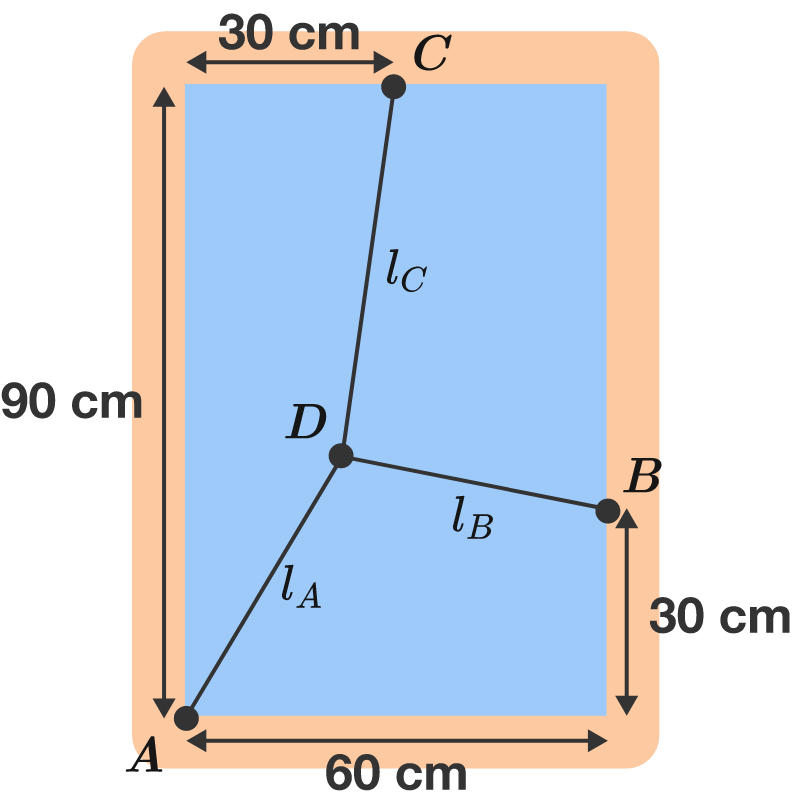# Triangular spider webIn this rectangular window frame, a spider begins to weave its web. The basic framework consists of three threads, which start from points $A, B,$ and $C$ and are glued together at point $D.$

What is the length $l_A$ of the first thread in centimeters?

Assumptions: Without tension, all threads have the same length, but they are stretched many times their original length. Thus, the tension force $F_i \approx - k l_i$ acting along a thread is approximately proportional to its total length $l_i$. While the lengths $l_i$ of the threads can be different, the spring constant $k$ is the same for all threads. Apart from the tension, no further forces act on the spider threads.

×